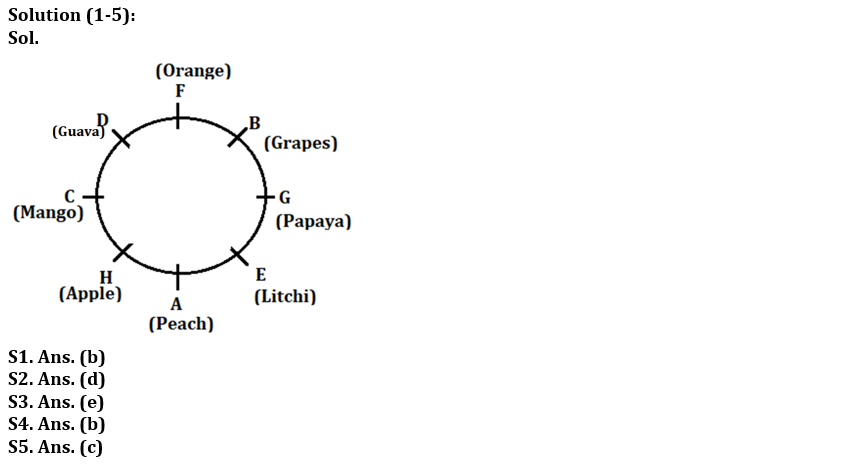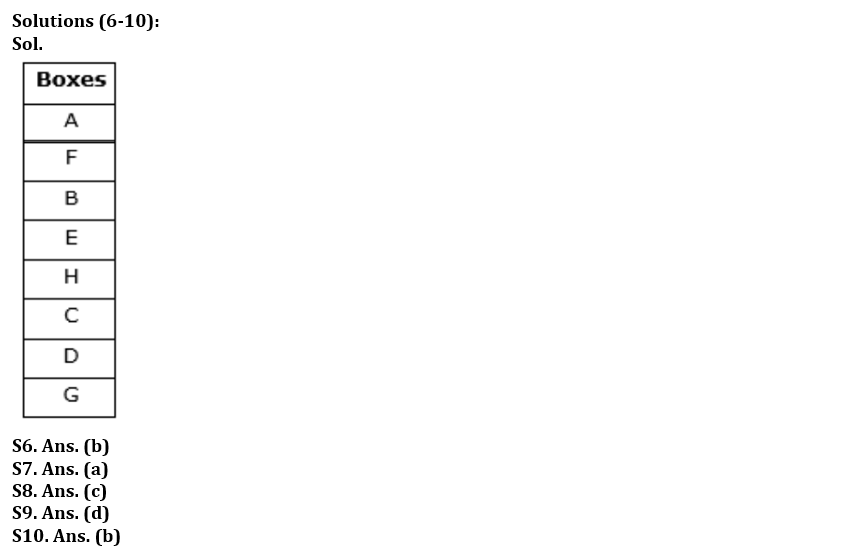Latest Banking jobs   »   Reasoning Ability Quiz For IBPS Clerk/PO...

# Reasoning Ability Quiz For IBPS Clerk/PO Prelims 2022- 14th August

Directions (1-5): Study the information carefully and answer the questions given below.
Eight persons A, B, C, D, E, F, G and H are sitting in a circular table and all of them facing towards center. They all like different fruits i.e. Grapes, papaya, Orange, Mango, Apple, Litchi, Guava and Peach but not necessary in same order.
A likes peach and sits second to the right of C. Two persons sit between C and E, who likes Litchi. B who likes Grapes sit opposite to H. B is not immediate neighbor of the one who likes peach. The one who likes Mango sits opposite to the one who likes Papaya. F sits second to the right of the one who likes papaya. D is immediate neighbor of the one who likes Orange. H does not like Guava. G is not immediate neighbor of A.

Q1. Which of the following fruit D likes?
(a) Apple
(b) Guava
(c) Orange
(d) Papaya
(e) None of these

Q2. Who among the following sits immediate left of F?
(a) D
(b) C
(c) G
(d) B
(e) None of these

Q3. Four of the following five are alike in certain way based from a group, find the one that does not belong to that group?
(a) C-G
(b) F-A
(c) B-H
(d) E-D
(e) D-H

Q4. Which of the following combination is true?
(a) B- Apple
(b) H-Apple
(c) D-Orange
(d) A-Litchi
(e) C-Papaya

Q5. Which among the following fruit G likes?
(a) Grapes
(b) Orange
(c) Papaya
(d) Mango
(e) None of these

Direction (6-10): Study the following data carefully and answer the questions accordingly.
Eight boxes i.e. A, B, C, D, E, F, G, and H are kept one above the other in a stack. The lowermost stack is numbered 1, the stack above it is numbered 2, and so on until the topmost stack is numbered 8. All the information is not necessarily in the same order. Three boxes are placed between Box A and Box H. Box B is places two places below Box A. Four Boxes are placed between Box B and Box G. Box D is placed in an even-numbered position below Box B. One box is placed between Box C and Box E. Box C is placed below Box H.

Q6. How many boxes are placed above Box F?
(a) Three
(b) One
(c) None
(d) More than three
(e) Two

Q7. How many boxes are placed between Box B and Box H?
(a) One
(b) Four
(c) Three
(d) None
(e) Two

Q8. How many boxes are placed below Box G?
(a) One
(b) Three
(c) None
(d) Two
(e) Four

Q9. Number of boxes placed above Box E is same as number of boxes placed below Box __.
(a) C
(b) D
(c) B
(d) H
(e) None of these

Q10. Which amongst the following box is placed in 3rd position from the bottom?
(a) H
(b) C
(c) E
(d) G
(e) None of these

Direction (11-15): In the following questions, a statement or statements are given followed by some conclusions. Study the following data carefully and answer the questions accordingly.

Q11. Statements: P > D ≥ E = T ≤ S < L ≤ A, B ≥ C > Z = H
Conclusions: I.E < A
II.P ≥ L
(a) If only conclusion I follows
(b) If only conclusion II follows
(c) If either conclusion I or II follows
(d) If neither conclusion I nor II follows
(e) If both conclusions I and II follow

Q12. Statements: E = P > C ≥ D = S ≤ R < L ≤ A, T ≥ C > Z = N
Conclusions: I. T = S
II. T > S
(a) If only conclusion I follows
(b) If only conclusion II follows
(c) If either conclusion I or II follows
(d) If neither conclusion I nor II follows
(e) If both conclusions I and II follow

Q13. Statements: B = M < Q ≤ Z ≥ S = T, Q = I > A
Conclusions: I. Z ≥ B
II. A < Z
(a) If only conclusion I follows
(b) If only conclusion II follows
(c) If either conclusion I or II follows
(d) If neither conclusion I nor II follows
(e) If both conclusions I and II follow

Q14. Statements: Z > T ≥ F, B ≥ I ≥ J = K > Z > M > N
Conclusions: I. B ≥ K
II. F < M
(a) If only conclusion I follows
(b) If only conclusion II follows
(c) If either conclusion I or II follows
(d) If neither conclusion I nor II follows
(e) If both conclusions I and II follows

Q15. Statements: B = H ≥ V < W > X > Y > Z, W = O > A < M
Conclusions: I. B > V
II. H ≥ X
(a) If only conclusion I follows
(b) If only conclusion II follows
(c) If either conclusion I or II follows
(d) If neither conclusion I nor II follows
(e) If both conclusions I and II follows

SolutionsS11. Ans. (a)
Sol. I.E < A – True
II. P ≥ L – False.

S12. Ans. (c)
Sol. I. T = S – False
II. T > S – False.

S13. Ans. (b)
Sol. I. Z ≥ B – False
II. A < Z – True.

S14. Ans. (a)
Sol. I. B ≥ K – True.
II. F < M – False.

S15, Ans.(d)
Sol. I. B > V – False
II. H ≥ X – False.#### Congratulations!Download Hindu Review of October 2021: Free PDF